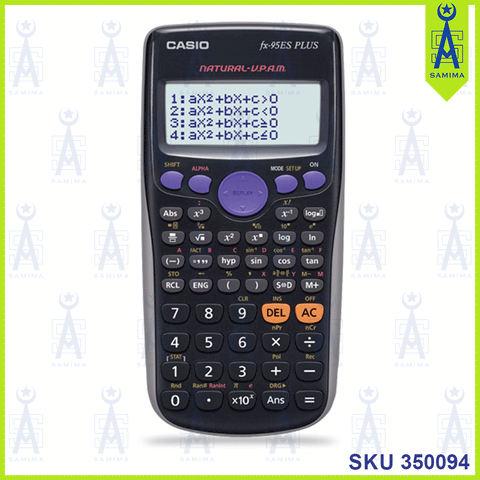# Software features

• ### equation mode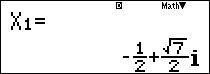• ### Inequality calculation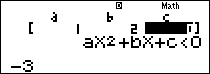• ### Prime factorization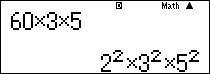• ### Ratio calculation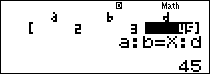• ### Random integer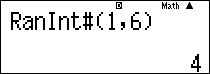## Other features

• One AAA-size(R03) battery
• 274 Functions
• Full Dot Display
• Plastic Keys
• Fraction calculation
• Combination and Permutation
• Logarithm log
• List-based STAT data editor
• Standard deviation
• Paired-variable statistics regression analysis
• Table function
• 9 variable memories
• Comes with new slide on hard case

We Also Recommend